Published: 15 February 2016

# Effect predictions of star pinion geometry phase adjustments on dynamic load sharing behaviors of differential face gear trains

Zhengminqing Li1
Wei Ye2
Linlin Zhang3
Rupeng Zhu4
1, 2, 3, 4Nanjing University of Aeronautics and Astronautics, Nanjing, China
Corresponding Author:
Zhengminqing Li
Views 67

#### Abstract

Face gear drives, which are suggested to be used in input stage gear drives of helicopter main gear boxes, are focused by many scholars. However, differential face gear trains, which can be employed in output stage gear drives of coaxial helicopter main gear boxes, are not to be addressed by researchers, and dynamic load sharing design solutions of differential face gear trains are yet to be investigated. Thus, in the study, a star pinion geometry phase adjustment solution, which is not to change drive ratios of differential face gear trains versus traditional geometry parameter adjustment solutions, is proposed, and a six DOF torsion dynamic model associated with four star pinions is established. Furthermore, dynamic load sharing behaviors of two version differential face gear trains are discussed, and the effects of star pinion geometry phase adjustments on two version differential face gear trains are predicted. The analytic results indicate the effects of the proposed star pinion geometry phase adjustments on dynamic load sharing behaviors of two version differential face gear trains are significant. These contributions would benefit to improve dynamic load sharing designs and engineering applications of differential face gear trains in the future.

## 2. Calculation solutions

### 2.1. Two version differential face gear trains

According to design requirements of helicopter main gear boxes, differential face gear trains are divided into two types. One is up-input version, namely, the first version, the other is down-input version, meaning, the second version. The sketches of two version differential face gear trains are shown in Fig. 1.

As illustrated in Fig. 1, in the first version differential face gear train, the input face gear is the up face gear, and the output face gear is the down face gear. While, in the second version differential face gear train, the input face gear is the down face gear, and the output face gear is the up face gear. Thus, the first version differential face gear train can be designed in main gear boxes associated with less depth, versus the second version.

Fig. 1Two version differential face gear trains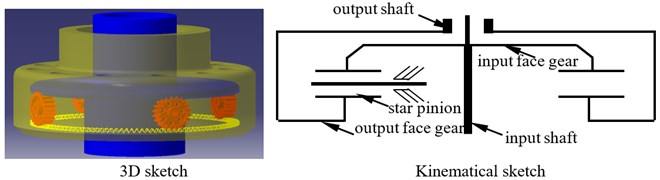a) The first version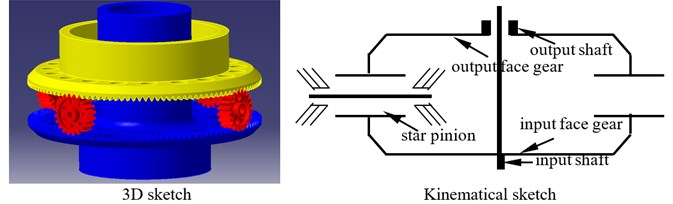b) The second version

### 2.2. Star pinion geometry phase calculation solution constructed

The star pinion geometry phase adjustment solution means getting the same geometry phases of star pinions at input stages by adjusting star pinion assembly positions. Thus, the star pinion geometry phase calculation solution is the base of the proposed star pinion geometry phase adjustment solution.

A face gear tooth is a kind of gear teeth associated with variable tooth thicknesses, and can be considered as a sequence in which modified involute gears are superimposed along its face width. Meanwhile, based on the contact viewpoints of face gear drives, face gear drives can be equivalent to involute gear drives. The evolution process of equivalent face gear drives is shown in Fig. 2.

As shown in Fig. 2, the contact relationship between face gears and pinions can be equivalent as that of between involute gears and pinions. Thus, star pinion geometry phases of differential face gear trains can be assumed as shown in Fig. 3.

Fig. 2An evolution process of equivalent face gear teeth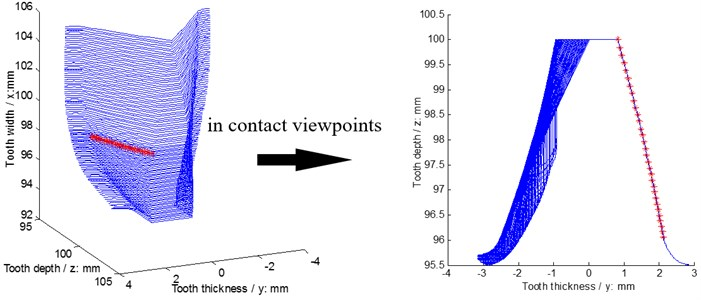Fig. 3A sketch of star pinion phases assumed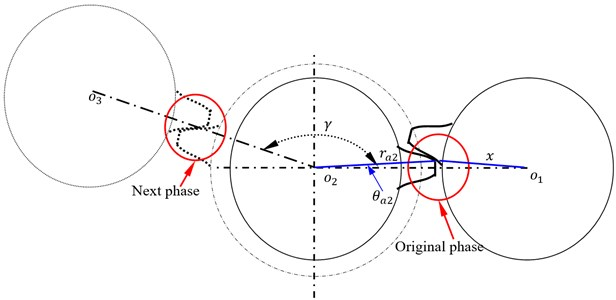In Fig. 3, $\gamma$ is defined as star pinion position angles, and is determined by design requirements of differential face gear trains, symbol $x$ can be derived by:

1
$x=\sqrt{{o}_{1}{o}_{2}^{2}+{r}_{a2}^{2}-2{o}_{1}{o}_{2}{r}_{a2}\mathrm{c}\mathrm{o}\mathrm{s}\left({\theta }_{a2}\right)},$

where ${r}_{a2}$ is an addendum radius of star pinions, and symbol ${\theta }_{a2}$ can be calculated by:

2
${\theta }_{a2}=\frac{\pi }{2{z}_{2}}+\left[\mathrm{t}\mathrm{a}\mathrm{n}\left(\alpha \right)-\alpha \right]-\left[\mathrm{t}\mathrm{a}\mathrm{n}\left({\alpha }_{a2}\right)-{\alpha }_{a2}\right],$

where ${z}_{2}$ is a tooth number of star pinions, $\alpha$ is a pressure angle of reference cycle of star pinions, ${\alpha }_{a2}$ is an addendum pressure angle of star pinions.

According to the relationship between symbol $x$ and the dedendum radius of driving face gear equivalent gears ${r}_{f1}$, original phases can be determined in a piecewise form as:

3
$original\mathrm{}\mathrm{}phase=\left\{\begin{array}{ll}0,& x={r}_{f1},\\ \left[\mathrm{t}\mathrm{a}\mathrm{n}\left({\alpha }_{x}\right)-{\alpha }_{x}\right]-\left[\mathrm{t}\mathrm{a}\mathrm{n}\left({\alpha }_{f1}\right)-{\alpha }_{f1}\right],& x>{r}_{f1},\end{array}\right\$

where ${\alpha }_{f1}$ is a dedendum pressure angle of driving face gears, and ${\alpha }_{x}$ can be expressed as:

4
${\alpha }_{x}=a\mathrm{c}\mathrm{o}\mathrm{s}\left(\frac{{r}_{b1}}{x}\right),$

where ${r}_{b1}$ is a base cycle radius of driving face gear equivalent gears.

Next phase deduced is similar with that of original phases, and can be derived in a piecewise form as:

5
$next\mathrm{}\mathrm{}phase=\left\{\begin{array}{ll}0,& {x}^{\mathrm{\text{'}}}={r}_{a3},\\ \left[\mathrm{t}\mathrm{a}\mathrm{n}\left({\alpha }_{a3}\right)-{\alpha }_{a3}\right]-\left[\mathrm{t}\mathrm{a}\mathrm{n}\left({\alpha }_{x}^{\text{'}}\right)-{\alpha }_{x}^{\text{'}}\right],& {x}^{\mathrm{\text{'}}}<{r}_{a3},\end{array}\right\$

where ${\alpha }_{a3}$ is an addendum pressure angle of driven face gears, and ${\alpha }_{x}^{\text{'}}$ can be written in:

6
${\alpha }_{x}^{\text{'}}=a\mathrm{c}\mathrm{o}\mathrm{s}\left(\frac{{r}_{b3}}{{x}^{\text{'}}}\right),$

where ${r}_{b3}$ is a base cycle radius of driven face gear equivalent gears, and ${x}^{\text{'}}$ can be given in a piecewise form as:

7
${x}^{\mathrm{\text{'}}}=\left\{\begin{array}{ll}\sqrt{{o}_{2}{o}_{3}^{2}+{r}_{f2}^{2}-2{o}_{2}{o}_{3}{r}_{f2}\mathrm{c}\mathrm{o}\mathrm{s}\left({\theta }_{f2}\right)}\mathrm{},& \mathrm{r}\mathrm{o}\mathrm{u}\mathrm{n}\mathrm{d}\left(\frac{\gamma {z}_{2}}{2\pi }\right)\frac{2\pi }{{z}_{2}}\ne {\theta }_{f2},\\ {o}_{2}{o}_{3}-{r}_{f2},& \mathrm{r}\mathrm{o}\mathrm{u}\mathrm{n}\mathrm{d}\left(\frac{\gamma {z}_{2}}{2\pi }\right)\frac{2\pi }{{z}_{2}}={\theta }_{f2},\end{array}\right\$

where ${r}_{f2}$ is a dedendum radius of driven face gear equivalent gears, symbol “round” means taking remainders, and symbol ${\theta }_{f2}$ can be obtained in a piecewise form as:

8
${\theta }_{f2}=\left\{\begin{array}{ll}\frac{\pi }{2{z}_{2}}+\left[\mathrm{t}\mathrm{a}\mathrm{n}\left(\alpha \right)-\alpha \right]-\left[\mathrm{t}\mathrm{a}\mathrm{n}\left({\alpha }_{f2}\right)-{\alpha }_{f2}\right],& \frac{\gamma {z}_{2}}{2\pi }=\mathrm{i}\mathrm{n}\mathrm{t},\\ \left\{\begin{array}{ll}\frac{\pi }{2{z}_{2}}+\left[\mathrm{t}\mathrm{a}\mathrm{n}\left(\alpha \right)-\alpha \right]-\left[\mathrm{t}\mathrm{a}\mathrm{n}\left({\alpha }_{f2}\right)-{\alpha }_{f2}\right],& {c}_{1}={c}_{2},\\ \frac{\pi }{2{z}_{2}}+\left[\mathrm{t}\mathrm{a}\mathrm{n}\left(\alpha \right)-\alpha \right]-\left[\mathrm{t}\mathrm{a}\mathrm{n}\left({\alpha }_{f2}\right)-{\alpha }_{f2}\right]-\mathrm{r}\mathrm{o}\mathrm{u}\mathrm{n}\mathrm{d}\left(\frac{\gamma {z}_{2}}{2\pi }\right)\frac{2\pi }{{z}_{2}},& {c}_{1}<{c}_{2},\\ \mathrm{r}\mathrm{o}\mathrm{u}\mathrm{n}\mathrm{d}\left(\frac{\gamma {z}_{2}}{2\pi }\right)\frac{2\pi }{{z}_{2}}-\frac{\pi }{2{z}_{2}}-\left[\mathrm{t}\mathrm{a}\mathrm{n}\left(\alpha \right)-\alpha \right]+\left[\mathrm{t}\mathrm{a}\mathrm{n}\left({\alpha }_{f2}\right)-{\alpha }_{f2}\right],& {c}_{1}>{c}_{2},\end{array}\right\& \begin{array}{c}\\ \frac{\gamma {z}_{2}}{2\pi }\ne \mathrm{i}\mathrm{n}\mathrm{t},\\ \end{array}\end{array}\right\$
${c}_{1}=\mathrm{r}\mathrm{o}\mathrm{u}\mathrm{n}\mathrm{d}\left(\frac{\gamma {z}_{2}}{2\pi }\right)\frac{2\pi }{{z}_{2}},\mathrm{}\mathrm{}\mathrm{}\mathrm{}{c}_{2}=\frac{\pi }{2{z}_{2}}+\left[\mathrm{t}\mathrm{a}\mathrm{n}\left(\alpha \right)-\alpha \right]-\left[\mathrm{t}\mathrm{a}\mathrm{n}\left({\alpha }_{f2}\right)-{\alpha }_{f2}\right],$

where symbol “int” means integers, ${\alpha }_{f2}$ is a dedendum pressure angle of star pinions.

### 2.3. Dynamic model

In engineering designs of coaxial helicopter main gear boxes associated with differential face gear trains, if traditional adjustment solutions of differential face gear trains, namely, tooth number adjustments, are employed, drive ratios of differential face gear trains would be changed, which would affect the total design of helicopter main gear boxes. While, the proposed star pinion geometry phase adjustment solution, meaning, assembly position adjustments of star pinions, would not change drive ratios and be implemented easily.

In order to evaluate influences of the proposed star pinion geometry phase adjustments on dynamic load sharing behaviors of two version differential face gear trains, a six DOF torsion dynamic model associated with four star pinions, which can reflect two versions by inputting base dynamic parameter adjustments, is formulated, as shown in Fig. 4.

As shown in Fig. 4, the total powers are transmitted to the input face gear, namely, the up face gear in the first version or the down face gear in the second version, as shown in Fig. 1, by the input shaft. Due to differential face gear trains, one part of powers on input face gear is consumed by external loads directly, and the rest part of powers is shared by star pinions through input face gear pairs. Then, the rest part of powers is collected to the output face gear, meaning, the down face gear in the first version or the up face gear in the second version, as shown in Fig. 1, by output facer gear pairs. Finally, the rest part of powers is used up by other out loads. In the power transmission procession of differential face gear trains, dynamic loads on star pinions determined by differential face gear train dynamics are different, which would affect differential face gear train performance greatly, due to star pinion geometry phases, etc. Thus, in order to assess star pinion dynamic load sharing behaviors, introducing star pinion geometry phases into static transmission errors and according to the dynamic model, as given in Fig. 4, the mathematic equations of the six DOF torsion dynamic model can be derived by:

9
$\left\{\begin{array}{l}{I}_{f}{\stackrel{¨}{\theta }}_{fl}+{c}_{m}{r}_{f}\left[4{r}_{f}{\stackrel{˙}{\theta }}_{fl}-{r}_{p}\left({\sum }_{i=4}{\stackrel{˙}{\theta }}_{pi}\right)-\left({\sum }_{i=4}{\stackrel{˙}{e}}_{i}\right)\right]\\ +{k}_{m}{r}_{f}\left[4{r}_{f}{\theta }_{fl}-{r}_{p}\left({\sum }_{i=4}{\theta }_{pi}\right)-\left({\sum }_{i=4}{e}_{i}\right)\right]={T}_{i}-{T}_{ol},\\ {I}_{p}{\stackrel{¨}{\theta }}_{p1}+{c}_{m}{r}_{p}\left[2{r}_{p}{\stackrel{˙}{\theta }}_{p1}-{r}_{f}\left({\stackrel{˙}{\theta }}_{fl}+{\stackrel{˙}{\theta }}_{fu}\right)-\left({\stackrel{˙}{e}}_{1}+{\stackrel{˙}{E}}_{1}\right)\right]\\ +{k}_{m}{r}_{p}\left[2{r}_{p}{\theta }_{p1}-{r}_{f}\left({\theta }_{fl}+{\theta }_{fu}\right)-\left({e}_{1}+{E}_{1}\right)\right]=0,\\ {I}_{p}{\stackrel{¨}{\theta }}_{p2}+{c}_{m}{r}_{p}\left[2{r}_{p}{\stackrel{˙}{\theta }}_{p2}-{r}_{f}\left({\stackrel{˙}{\theta }}_{fl}+{\stackrel{˙}{\theta }}_{fu}\right)-\left({\stackrel{˙}{e}}_{2}+{\stackrel{˙}{E}}_{2}\right)\right]\\ +{k}_{m}{r}_{p}\left[2{r}_{p}{\theta }_{p2}-{r}_{f}\left({\theta }_{fl}+{\theta }_{fu}\right)-\left({e}_{2}+{E}_{2}\right)\right]=0,\\ {I}_{p}{\stackrel{¨}{\theta }}_{p3}+{c}_{m}{r}_{p}\left[2{r}_{p}{\stackrel{˙}{\theta }}_{p3}-{r}_{f}\left({\stackrel{˙}{\theta }}_{fl}+{\stackrel{˙}{\theta }}_{fu}\right)-\left({\stackrel{˙}{e}}_{3}+{\stackrel{˙}{E}}_{3}\right)\right]\\ +{k}_{m}{r}_{p}\left[2{r}_{p}{\theta }_{p3}-{r}_{f}\left({\theta }_{fl}+{\theta }_{fu}\right)-\left({e}_{3}+{E}_{3}\right)\right]=0,\\ {I}_{p}{\stackrel{¨}{\theta }}_{p4}+{c}_{m}{r}_{p}\left[2{r}_{p}{\stackrel{˙}{\theta }}_{p4}-{r}_{f}\left({\stackrel{˙}{\theta }}_{fl}+{\stackrel{˙}{\theta }}_{fu}\right)-\left({\stackrel{˙}{e}}_{4}+{\stackrel{˙}{E}}_{4}\right)\right]\\ +{k}_{m}{r}_{p}\left[2{r}_{p}{\theta }_{p4}-{r}_{f}\left({\theta }_{fl}+{\theta }_{fu}\right)-\left({e}_{4}+{E}_{4}\right)\right]=0,\\ {I}_{f}{\stackrel{¨}{\theta }}_{fu}+{c}_{m}{r}_{f}\left[4{r}_{f}{\stackrel{˙}{\theta }}_{fu}-{r}_{p}\left({\sum }_{i=4}{\stackrel{˙}{\theta }}_{pi}\right)+\left({\sum }_{i=4}{\stackrel{˙}{E}}_{i}\right)\right]\\ +{k}_{m}{r}_{f}\left[4{r}_{f}{\theta }_{fu}-{r}_{p}\left({\sum }_{i=4}{\theta }_{pi}\right)+\left({\sum }_{i=4}{E}_{i}\right)\right]=-{T}_{ou},\end{array}\right\$

where subscript $p$ and $f$ express a star pinion and a face gear respectively, subscript $fl$ and $fu$ are a down face gear and a up face gear respectively, $\theta$ is a torsion degree of freedom, $I$ is a moment of inertia, ${T}_{i}$ is a input torsion, ${T}_{ol}$ and ${T}_{ou}$ are output torsions on the down and up face gears respectively, “.” is first derivative, “.." is second derivative, $r$ is a base circle radius, ${k}_{m}$ is mesh stiffness, ${c}_{m}$ is mesh damping, $e$ and $E$ are STE of the down and up face gear drives, respectively.

In addition, the input or output stage dynamic load sharing coefficient can be defined as:

10
${D}_{i/o}=\sqrt{\frac{{\sum }_{i=1}^{{N}_{F}}{A}_{Fi}^{2}}{{N}_{F}}},$

and the total dynamic load sharing coefficient $D$ can be determined by:

11
$D=\sqrt{\frac{{D}_{i}^{2}+{D}_{o}^{2}}{2}},$

where ${A}_{Fi}$ is an amplitude versus frequencies, ${N}_{F}$ is a number of frequencies, subscript $i$ and $o$ express input and output dynamic load sharing coefficients, respectively.

Fig. 4A torsion dynamic model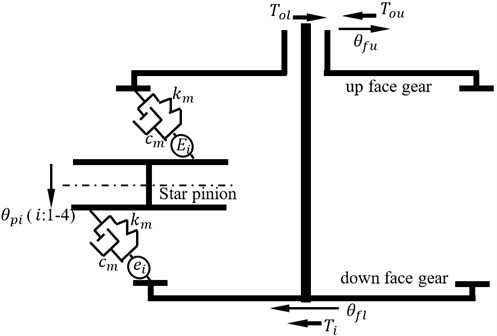## 3. Simulations and effect predictions

### 3.1. Simulations

In order to evaluate dynamic load sharing behaviors of two version differential face gear trains without star pinion geometry phase adjustments, namely, star pinion equispaced, as shown in Fig. 5, geometric parameters, operating conditions and material characteristics of an example case of two versions associated with four star pinions are given in Table 1.

Fig. 5A sketch of star pinion equispaced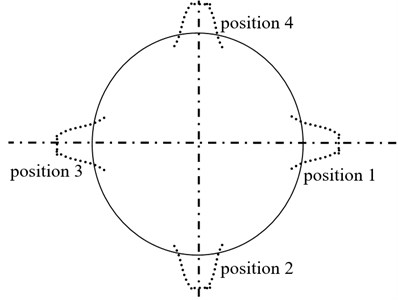In the case of Fig. 5, according to the parameters listed in Table 1 and the proposed star pinion geometry phase calculation solutions, the star pinion geometry phases of the example case of two versions are calculated as given in Table 2.

According to Eq. (9), the dynamic load sharing behaviors of the example case of two version differential face gear trains are simulated as shown in Figs. 6, 7.

Table 1Parameters of the example case

 Names Values Units Geometric parameters Modulus 4 mm Pressure angle 25 ° Tooth number of pinions 25 – Tooth number of face gears 105 – Addendum coefficient 1 – Clearance coefficient 0.25 – Operating conditions Power 80 kW Input rotation speed 400 r/min Material characteristics Modulus of elasticity 210000 MPa Poisson ratio 0.3 –

Fig. 6The dynamic load sharing behaviors of the first version simulated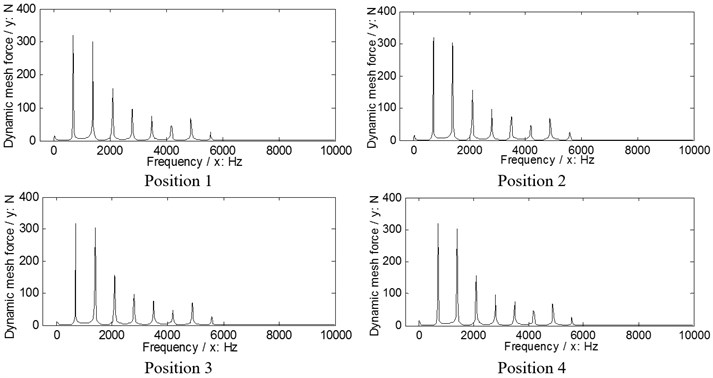a) Input stages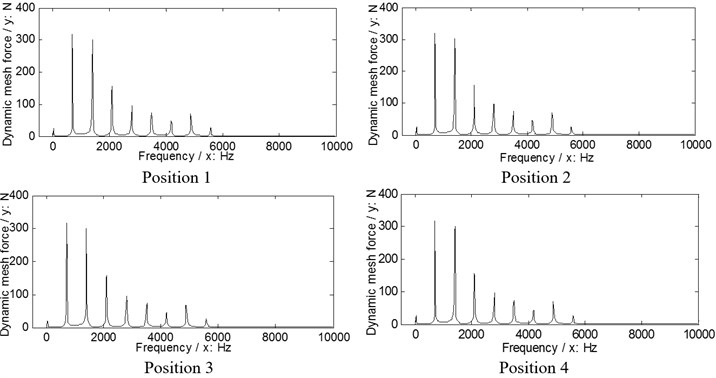b) Output stages

According to the results in Figs. 6, 7 and Eq. (10) as well as Eq. (11), the dynamic load sharing coefficients of the example case of two versions without star pinion geometry phase adjustments can be calculated as listed in Table 3.

According to the results as listed in Table 3, the total dynamic load sharing coefficient of the second version is better than that of the first version, but the dynamic load sharing behaviors of two versions are not well due to the results beyond the normal range suggested by design experiences, namely, 1.02 to 1.06.

Table 2Star pinion geometry phases of the example case of two versions

 Types Position 1 Position 2 Position 3 Position 4 Unit Output stages of the first and second versions 0.0045 0.0188 0.0155 0.0188 rad Input stages of the first and second versions 0.002 0.0052 0.0085 0.0052

 The first version The second version Coefficient of input stages 1.23 1.14 Coefficient of output stages 1.07 1.04 Total coefficient 1.15 1.09

Fig. 7The dynamic load sharing behaviors of the second version simulated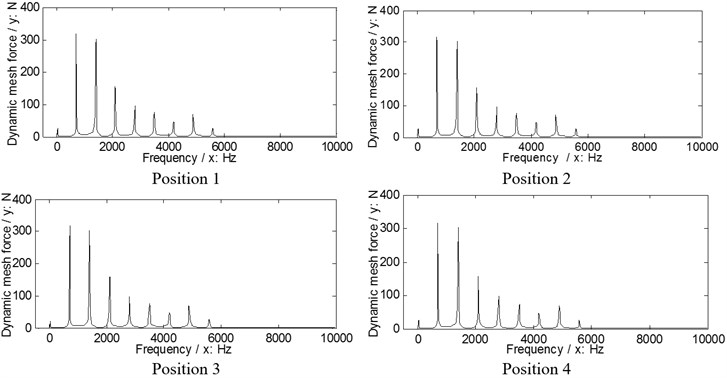a) Input stages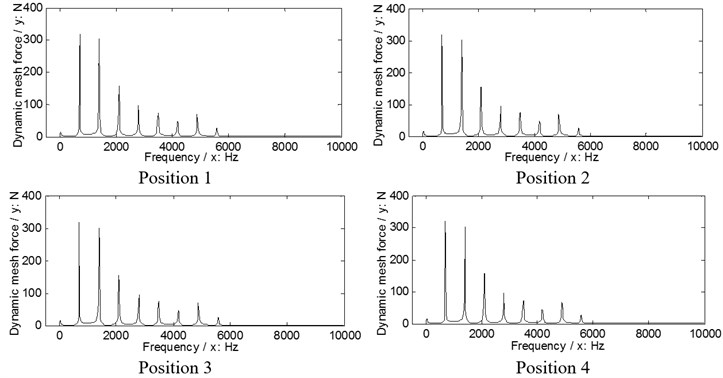b) Output stages

### 3.2. Effect predictions

In order to assess effects of the proposed star pinion geometry phase adjustments on dynamic load sharing behaviors of two versions, the star pinion positions adjusted of the example case, which would cause the same geometry phases of star pinions at input stages, is calculated as shown in Fig. 8, and the star pinion geometry phases of the example case associated with the adjustments are obtained as listed in Table 4.

Fig. 8A sketch of star pinion assembly position adjustments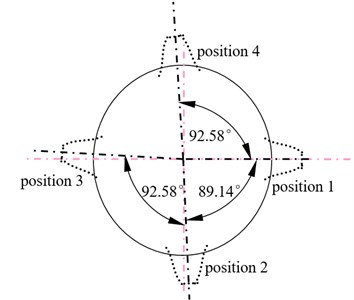Fig. 9The dynamic load sharing behaviors of the first version associated with the proposed adjustments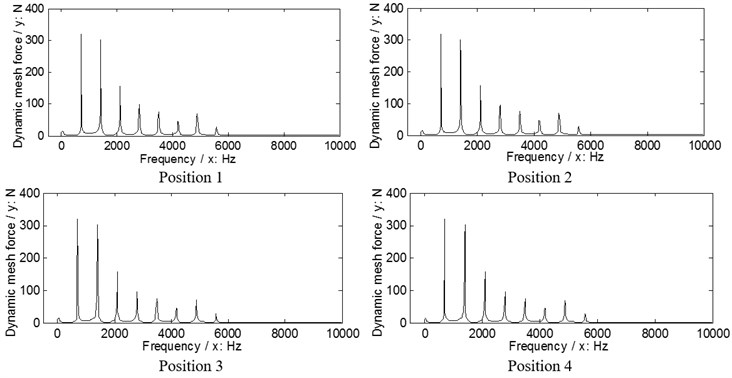a) Input stages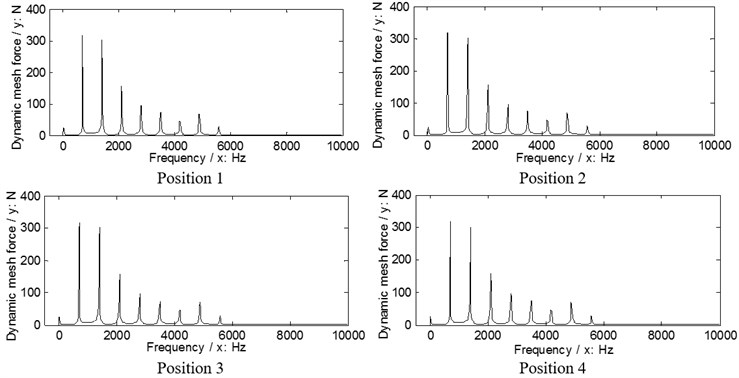b) Output stages

Fig. 10The dynamic load sharing behaviors of the second version associated with the proposed adjustments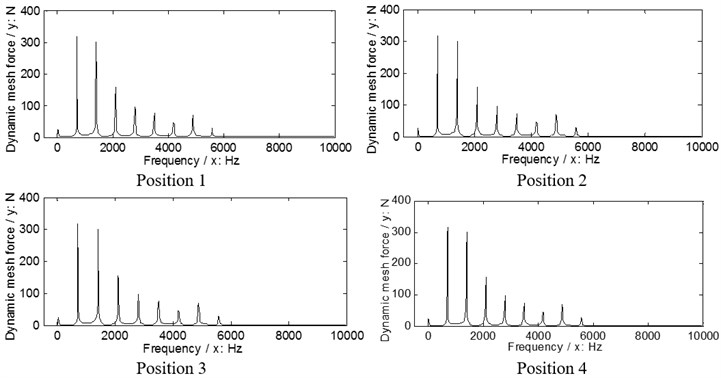a) Input stages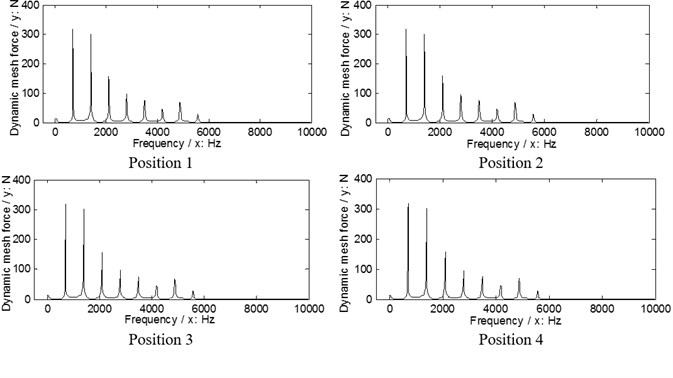b) Output stages

According to Eq. (9), the dynamic load sharing behaviors of the example case of two versions associated with the proposed star pinion geometry phase adjustments are simulated as shown in Figs. 9, 10.

According to the results in Figs. 9. 10, the dynamic load sharing coefficients of the example case of two versions associated with star pinion geometry phase adjustments can be calculated as listed in Table 5.

Table 4Geometry phases of the example case of two versions associated with the proposed adjustments

 Types Position 1 Position 2 Position 3 Position 4 Unit Output stages of the first and second versions 0.0045 0.022 0.022 0.022 rad Input stages of the first and second versions 0.002 0.002 0.002 0.002

Table 5Dynamic load sharing coefficients associated with star pinion geometry phase adjustments

 The first version The second version Coefficient of input stages 1.032 1.03 Coefficient of output stages 1.019 1.02 Total coefficient 1.026 1.025

The difference between before and after star pinion geometry phase adjustments can be defined as:

12
$\eta =\frac{{D}_{old}-{D}_{ad}}{{D}_{old}}×100%,$

where ${D}_{old}$ is a total coefficient without the proposed star pinion geometry phase adjustment, ${D}_{ad}$ is a total coefficient with the proposed star pinion geometry phase adjustment.

According to the results as listed in Table 5 and Table 3, and Eq. (12), the differences can be calculated as listed in Table 6.

According to Table 6, the effects of star pinion geometry phase adjustments on dynamic load sharing behaviors are significant, and the improved effect of the first version is better than that of the second version.

Table 6The differences between before and after adjustments

 The first version The second version 10.8 % 5.96 %

## 4. Conclusions

In the study, three conclusions can be extracted as follows:

1) A star pinion geometry phase adjustment solution, which would not change drive ratios of differential face gear trains versus traditional parameter adjustment solutions, is proposed, and a star pinion geometry phase calculation solution is constructed.

2) Dynamic load sharing behaviors of the first version differential face gear train is worse than those of the second version under the condition of star pinion equispaced, namely, without the proposed star pinion geometry phase adjustment.

3) The effects of the proposed star pinion geometry phase adjustment solution on dynamic load sharing behaviors of two version differential face gear trains are significant, and the improved effects of the first version associated with the proposed adjustment solution is better than that of the second version.

These contributions would be helpful to improve dynamic load sharing designs of differential face gear trains in the future.

18 October 2015
Accepted
27 December 2015
Published
15 February 2016
SUBJECTS
Mechanical vibrations and applications
Keywords
differential face gear trains